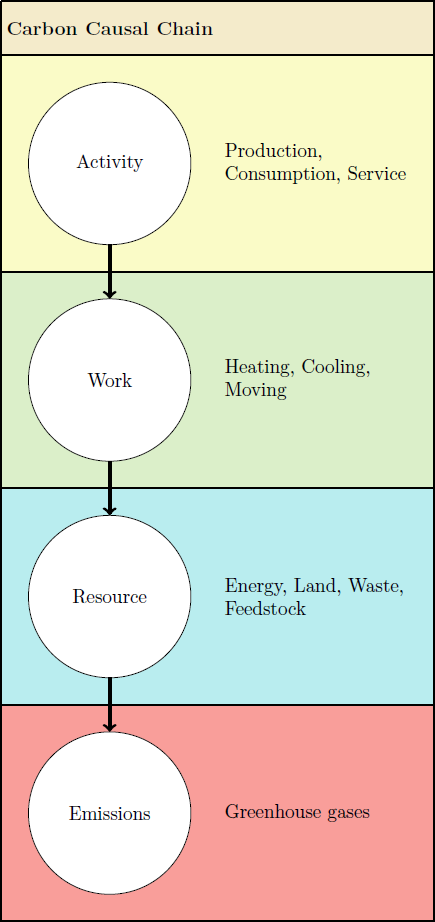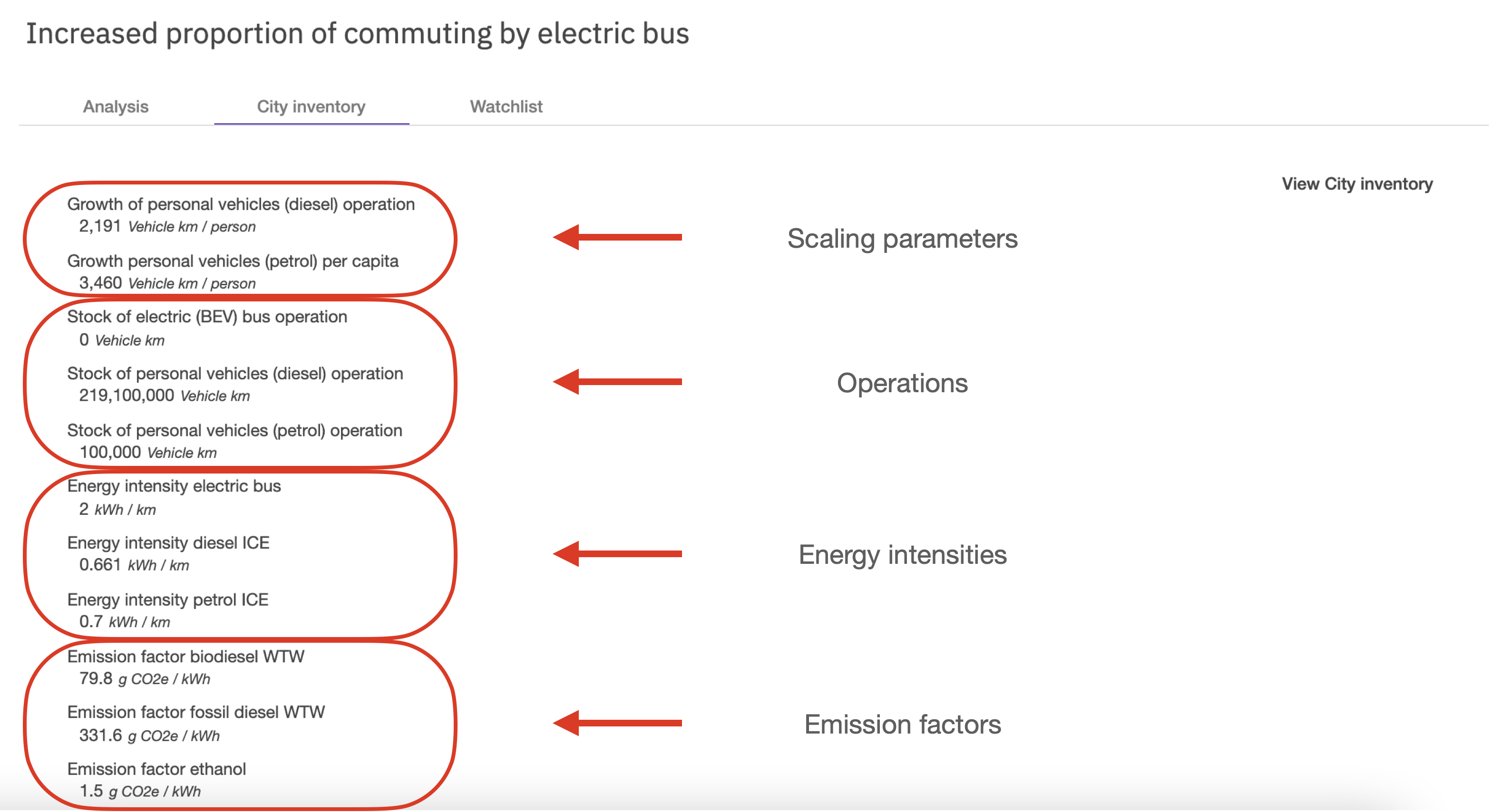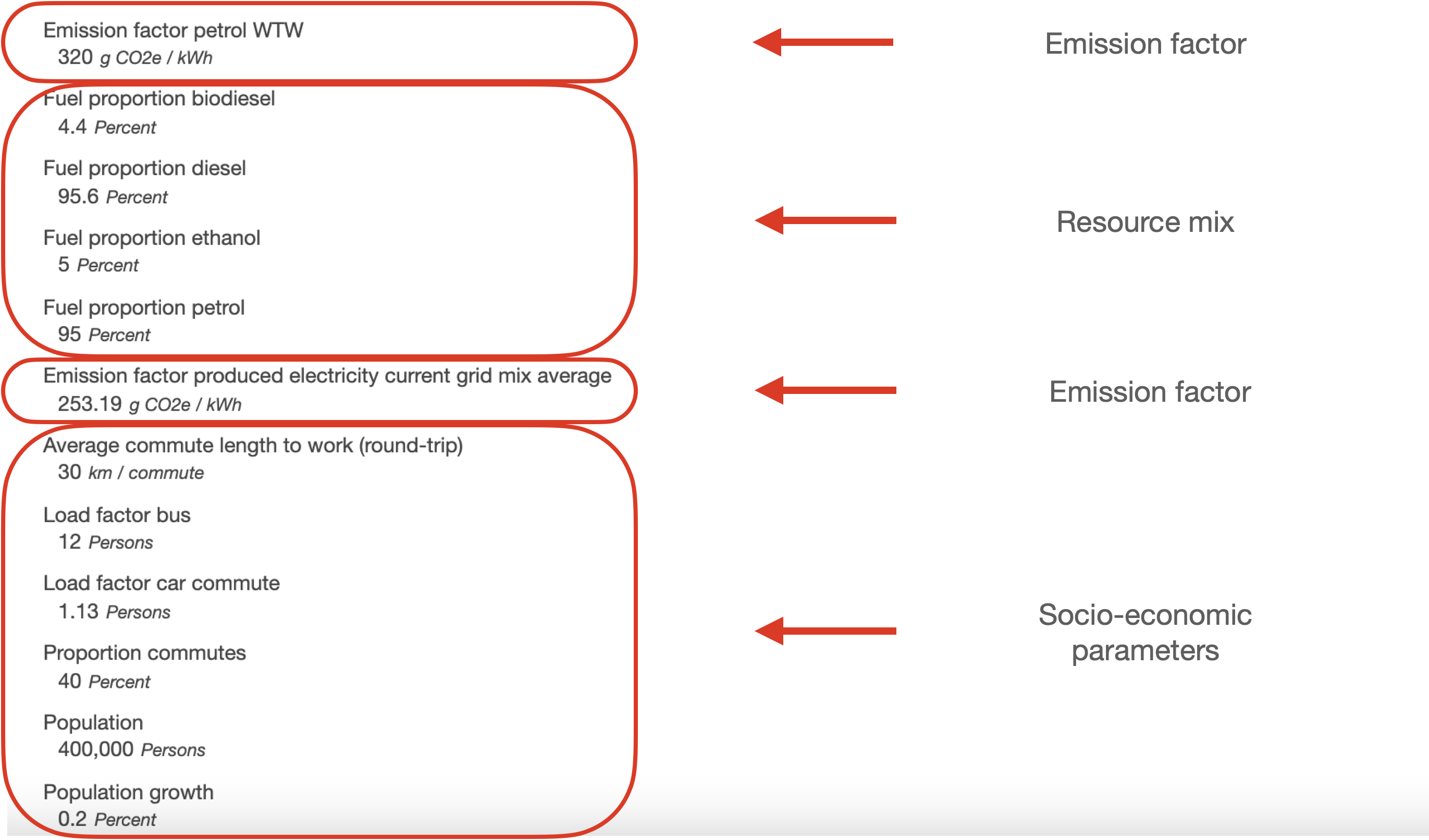# Connection between parameters and Transition Elements

The Transition elements are based on the Carbon Causal Chains. The data used to calculate each Carbon Causal Chain of a mechanism, and therefore the carbon abatement of a Transition element, can be found in the City inventory under the Inventory tab.

Recap: Carbon Causal Chains

A Carbon Causal Chain (CCC) is a logic model for the calculation of the total amount of emissions from the operations by which activities are carried out. The CCCs consist of four distinct but connected levels. These levels are classified as: activity, work, resource and emission (Fig. 1). The CCC describes the causal flow from an activity to an emission.Fig. 1

The levels outlined in the CCC can be expressed as followed:

• Activity - the actual number of operations carried out with a particular mechanism and resource to satisfy a city's need (i.e. kilometres driven by petrol cars to fulfill the need to travel).
• Work - the amount of energy needed in kWh to carry out one unit of the operations with a particular mechanism and resource. This is what is called the energy intensity of the operation (i.e. the amount of kWh needed to drive one kilometre with petrol cars).
• Resource - the total amount of energy in kWh needed to carry out all the operations with a particular mechanism and resource to serve a need (i.e. the total amount of energy in kWh needed to drive all the kilometres with petrol cars that fulfill the need to travel). This is the result of multiplying the number of operations with the corresponding energy intensity for the resource used.
• Emissions - applying the correct emission factor (g CO2eq/kWh) for a particular resource to the total amount of energy needed to carry out all the operations, results in the total amount of greenhouse gas emissions coming from the activity.

Example:

In ClimateVille, petrol cars are driving 100 km per year to serve the need to travel. Here, the 100 km is the actual number of operations carried out. The mechanism used for those operations are cars which use the resource petrol to operate. In order to drive one of those 100 km with petrol cars, a certain amount of energy is needed, the energy intensity. For the sake of the example, let's assume that in order to drive one kilometre with petrol cars, 0.7 kWh are used. Multiplying the operations (100 km) with the energy intensity for petrol cars (0.7 kWh), results in the total amount of energy used to carry out all the operations (100 km x 0.7 kWh = 70 kWh). Now we can multiply to correct emission factor for the resource used (petrol: 320 g CO2eq/kWh) with the total amount of energy (70 kWh), to calculate the total amount of greenhouse gas emissions resulting from carrying out all the operations (70 kWh x 320 g CO2eq = 22400 g CO2eq or 22.4 kg CO2eq).Fig. 2

Let's take the example of shifting trips being carried out by fossil-fuelled cars to electric busses which the Transition element Increased proportion of commuting by electric bus represents. This Transition element is pulling the data from the CCCs for fossil-fuelled cars (i.e. diesel and petrol cars) and electric busses, their scaling parameters as well as any socio-economic parameters connected to those mechanisms from your City inventory. You can see these data points in the City inventory tab of any Transition element (see Fig. 2 and 3).Fig. 2Fig. 3

Simply put, the Transition element is comparing the CCCs of different mechanisms with each other in order to determine the total emissions resulting from any mechanism.

By adjusting the target value for each Transition element, i.e. how many trips should be carried out by electric buses by the city's target year instead of with fossil-fuelled cars, the Transition element can calculate the carbon abatement achieved through the shift.